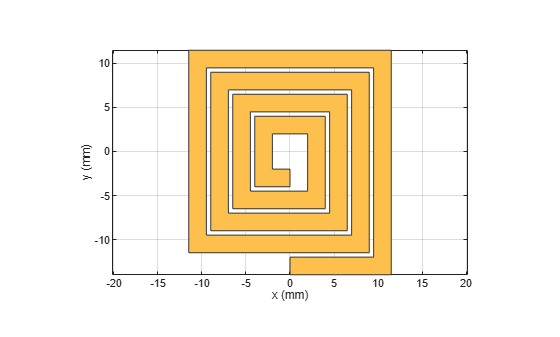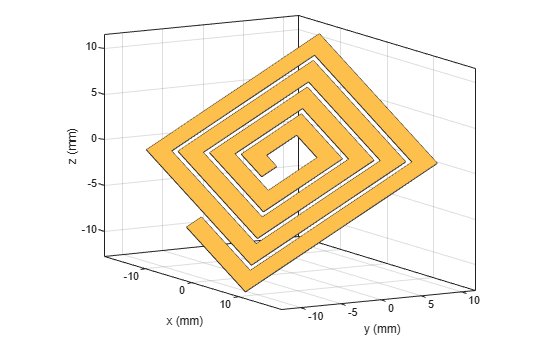# rotate

Rotate RF PCB shape about defined axis

Since R2021b

## Syntax

``c = rotate(shape,angle,axis1,axis2)``

## Description

example

````c = rotate(shape,angle,axis1,axis2)` rotates a shape by a specified angle about an axes defined by two points `axis1` and `axis2`.```

## Examples

collapse all

Create and display a spiral trace.

```trace = traceSpiral; show(trace)```Specify two points that define the axes of rotation.

```pt1 = [0 0 0]; pt2 = [1 1 0];```

Rotate the spiral trace by 45 degrees about the axis.

`traceRot = rotate(trace,45,pt1,pt2);`

Display the rotated shape. Set the camera line of sight to display in 3-D space.

```show(traceRot) view(60,10)```## Input Arguments

collapse all

RF PCB shape created using custom elements and shape objects of RF PCB Toolbox™, specified as an object.

Example: `shape = bendCurved;` specifies the shape as a `bendCurved` object.

Angle of rotation, specified as a scalar in degrees.

Example: `45` rotates the shape around the axis by 45 degrees.

Data Types: `double`

One point on the axis of rotation, specified as a three-element vector of Cartesian coordinates in meters.

Example: `[0 0 0]`

Data Types: `double`

Second point on the axis of rotation, specified as a three-element vectors of Cartesian coordinates in meters. `axis2` must be different than `axis1`.

Example: `[0 0 1]`

Data Types: `double`

## Version History

Introduced in R2021b